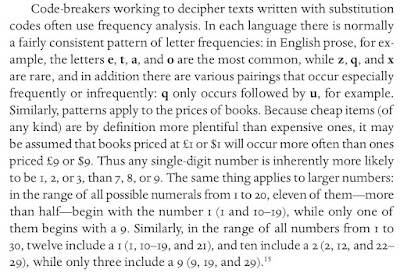## Saturday, 7 September 2019

### The Brölemann Price-Code: A Partial Decipherment?

A recent post caused me to look again at the question of the price-code use by at least one of the Brölemanns, and found in their manuscripts.

In my much older post about the Brölemann catalogues, I wrote that If enough examples could be collected, it ought also to be possible to decipher the Brölemann price-code. From the images we have, it is apparent that x=0, and other numbers are represented by cd,  l, q, s, t, and u.

Looking at all the coded prices in that post, we find the following encoded prices: dcx, uc, ufx, qxx, udx, fc, ucx, dxx, lxx, txx, ufx, fc, and uux, which looks like gibberish.

If we re-arrange them alphabetically, however, with two-digit codes before three-digit ones, some patterns become clearer:
fc
fc
uc

dcx
dxx

lxx

qxx

txx

udx
ucx
ufx
ufx
uux

First, it is now clearer that there are only three two-digit numbers, and of these, two of them begin with f. This is likely to represent a high number, such as 8 or 9: most manuscripts cost a three-digit amount, so a two-digit amount is more likely to be something like 85 or 90 than, say, 15 or 20.

Second, the last digit is always x (doubtless representing 0), except in two-digit numbers, which all end with c, which therefore likely represents 5, if the currency was decimal.

Third, for three-digit numbers, the most common first letter is u (occurring 5 times) and the next most frequent letter is d (occurring twice). As I wrote in my chapter in Ian Jackson's book about price-codes:[click to enlarge]
Following this logic, the Brölemann code-letters u and d ought to represent the numbers 1 and 2, and we have already surmised above that c = 5.  We already know that other letters include s and t.

This suggests that the Brölemann code may have been based on a very simple nmemonic, in which u = un, d = deux, = trois, q = quatre, c = cinq, and s = six / sept. The l and f that occur are currently unexplained by such a simple code, but the study of more books with the Brölemann price-code should clarify the situation.